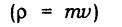Force and Laws of Motion Class 9 Notes – Here We have provided summary and revision notes for Class 9 Science Chapter 9. This CBSE notes contains CBSE Key Notes, CBSE Revision Notes, Short Key Notes, images, diagrams of the complete Chapter 9 titled Force and Laws of Motion of Science taught in class 9. If you are a student of class 9 who is using NCERT Textbook to study Science, then you must come across Chapter 9 Force and Laws of Motion. After you have studied lesson, you must be looking for notes to memorize. Here you can get complete Chapter 9 Force and Laws of Motion class 9 notes in one place. For a better understanding of this chapter, you should also see NCERT Solutions for Class 9 Science Chapter 9 Force and Laws of Motion.

CBSE Class 9 Science Notes Chapter 9 Force and Laws of Motion Pdf free download is part of Class 9 Science Notes for Quick Revision. Here we have given NCERT Class 9 Science Notes Chapter 9 Force and Laws of Motion. https://meritbatch.com/force-laws-motion-cbse-notes-class-9-science/

## CBSE Class 9 Science Notes Chapter 9 Force and Laws of Motion

Force And Laws Of Motion Class 9 Notes
Facts that Matter

Force: It is a push or pulls on an object that produces an acceleration in the body on which it acts. S.I. unit of force is Newton.

A force can do three things on a body.

• It can change the speed of the body.
• It can change the direction of motion of a body.
• It can change the shape of a body.

Balanced forces: Forces are said to be balanced forces if they nullify one another and their resultant force is zero.Frictional force: The force that always opposes the motion of objects is called a force of friction.

Class 9 Force And Laws Of Motion Notes
The second law of motion:
The rate of change of momentum of an object is proportional to the applied unbalanced force in the direction of the force. Mathematically,Momentum: The momentum of an object is the product of its mass and velocity and has the same direction as that of the velocity. Its S.I. unit is kg m/s.Force And Laws Of Motion Class 9 Notes Pdf
1 Newton:
A force of one Newton produces an acceleration of 1 m/s2 on an object of mass 1 kg.
IN. = 1 kg m/s2 (F = ma)

Third law of motion: To every action, there is an equal and opposite reaction and they act on two different bodies.

Conservation of momentum: If the external force on a system is zero, the momentum of the system remains constant i.e., in an isolated system, the total momentum remains conserved.Suppose A and B are two balls, they have mass mA and and initial velocities uA and uB as shown in above figure before collision. The two bodies collide and force is exerted by each body. There is change in their velocities due to collision.

Unbalanced forces: When two opposite forces acting on a body, move a body in the direction of the greater force or forces which brings motion in a body are called as unbalanced forces.

First law of motion: An object remains in a state of rest or of uniform motion in a straight line unless acted upon by an external unbalanced force.

Class 9 Chapter 9 Science Notes
Inertia:
The natural tendency of an object to resist a change in their state of rest or of uniform motion is called inertia.
The mass of an object is a measure of its inertia.
Its S.I. unit is kg.
A body with greater mass has greater inertia.
(mAuA + mAuB) is the total momentum of the two balls A and B before collision and (mAvA + mBvB) is their total momentum after the collision.
The sum of momenta of the two objects before collision is equal to the sum of momentum after the collision, provided there is no external unbalanced force acting on them.
This is known as the law of conservation of momentum.

We hope the given CBSE Class 9 Science Notes Chapter 9 Force and Laws of Motion Pdf free download will help you. If you have any query regarding NCERT Class 9 Science Notes Chapter 9 Force and Laws of Motion, drop a comment below and we will get back to you at the earliest.